Courses

# Test: 35 Year JEE Previous Year Questions: Organic Chemistry - Some Basic Principles & Technique

## 54 Questions MCQ Test Chemistry 35 Years JEE Main & Advanced Past year Papers | Test: 35 Year JEE Previous Year Questions: Organic Chemistry - Some Basic Principles & Technique

Description
This mock test of Test: 35 Year JEE Previous Year Questions: Organic Chemistry - Some Basic Principles & Technique for JEE helps you for every JEE entrance exam. This contains 54 Multiple Choice Questions for JEE Test: 35 Year JEE Previous Year Questions: Organic Chemistry - Some Basic Principles & Technique (mcq) to study with solutions a complete question bank. The solved questions answers in this Test: 35 Year JEE Previous Year Questions: Organic Chemistry - Some Basic Principles & Technique quiz give you a good mix of easy questions and tough questions. JEE students definitely take this Test: 35 Year JEE Previous Year Questions: Organic Chemistry - Some Basic Principles & Technique exercise for a better result in the exam. You can find other Test: 35 Year JEE Previous Year Questions: Organic Chemistry - Some Basic Principles & Technique extra questions, long questions & short questions for JEE on EduRev as well by searching above.
QUESTION: 1

### Read the following Statement-1(Asseration)  and Statement -2 (Reason) and answer as per the options given below : Q.  Statement -1: Aryl halides undergo nucleophilic substitution with ease. Statement -2:The carbon-halogen bond in aryl halides has partial double bond character.

Solution:

Statement -1 is false beca use aryl halides do not undergo nucleophilic substitution under ordinary conditions. This is due to resonance, because of which the carbon–chlorine bond acquires partial double bond character, hence it becomes shorter and stronger and thus cannot be replaced by nucleophiles.

QUESTION: 2

### Statement -1: Phenol is more reactive than benzene towards electrophilic substitution reactions. Statement-2:In the case of phenol, the intermediate carbocation is  more resonance stabilized.

Solution: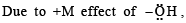its in termediate carbocation is more stable than the one in benzene.

QUESTION: 3

### Statement -1: Molecules that are not superimpossable on their mirror images are chiral. Statement -2: All chiral molecules have chiral centres.

Solution:

Statement-1 is correct. Statement-2 is incorrect because compound can be chiral even in the absence of chiral atoms.

QUESTION: 4

Arrangement of (CH3)3C –, (CH3)2CH –, CH3 – CH2 – when attached to benzyl or an unsaturated group in increasing order of inductive effect is

Solution:

–CH3 group has + I effect , as number of –CH3 group increases, the inductive effect increases.
Therefore the correct order is

CH3– CH2– < (CH3)2CH– < (CH3)3C –

QUESTION: 5

A similarity between optical and geometrical isomerism is that

Solution:

Sterioisomerism involve those isomers which contain same ligands in their co-ordination spheres but differ in the arrangement of these ligands in space. Stereoisomerism is of two type geomerical isomerism and optical isomerism. In geomerical isomerism ligands occupy different positions around the central metal atom or ion.

NOTE : In optical isomerism isomers have same formula but differ in their ability to rotate directions of the plane of polarised light.

QUESTION: 6

Which of the following does not show geometrical isomerism?

Solution: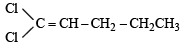does not show geometrical isomerism due to presence of two similar Cl atoms on the same C-atom. Geometrical isomerism is shown by compounds in which the groups/atoms attached to C = C are different.

QUESTION: 7

The functional group, which is found in amino acid is

Solution:

Amino acids contain – NH2 and – COOH groups e.g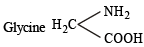QUESTION: 8

Which of the following compounds has wrong IUPAC name?

Solution:

The correct name is 3 - methylbut - 2 - ol

QUESTION: 9

The IUPAC name of CH3COCH(CH3), is

Solution: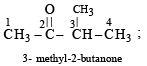QUESTION: 10

In which of the following species is the underlined carbon having sp3 hybridisation?

Solution:

In molecules (a), (c) and (d), the carbon atom has a multiple bond, only (b) has sp3 hybridization.

QUESTION: 11

Racemic mixture is formed by mixing two

Solution:

A mixture of equal amount of two enantiomers is called a racemic mixture. A racemic mixture does not rotate plane– polarized light. They are optically inactive because for every molecule in a racemic mixture that rotate plane of polarized light in one direction, there is a mirror image molecule that rotates the plane in oppsite direction.

QUESTION: 12

Following types of compounds (as I, II)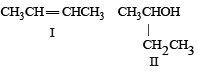are studied in terms of isomerism in:

Solution:

TIPS/Formulae : Stereoisomerism is of two types i.e., geometrical isomerism and optical isomerism
Both the structures shows sterioisomerism. Structure I shows geometrical isomerism as it contains two different atoms(H) and groups (CH3) attached to each carbon containing double bond.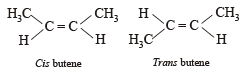Structure II shows optical isomerism as it contains a chiral carbon (attached to four different group) atom.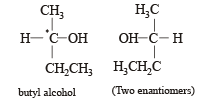QUESTION: 13

The reaction: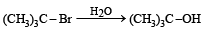Solution:

The hydrolysis of t-butyl bromide is an example of SN1 reaction. The reaction consists of two steps.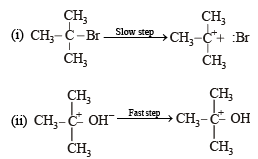QUESTION: 14

In the anion HCOO the two carbon-oxygen bonds are found to be of equal length. what is the reason for it ?

Solution:

HCOO exists in following resonating structures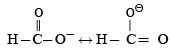Hence in it both the carbon oxygen bonds are found equal.

QUESTION: 15

The general formula CnH2nO2 could be for open chain

Solution:

CnH2nO2 is general formula for carboxylic acid

QUESTION: 16

Among the following four structures I to IV,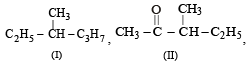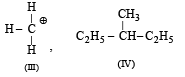it is true that

Solution: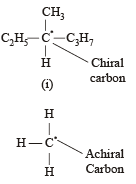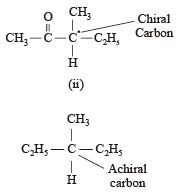QUESTION: 17

Which one of the following has the minimum boiling point ?

Solution:

NOTE : Among isomeric alkanes, the straight ch ain isomer has higher boiling point than the branched chain isomer. The greater the branching of the chain, the lower is the boiling point. Further due to the presence of π electrons, these moleculs are slightly polar and hence have higher boiling points than the corrosponding alkanes.
Thus B.pt. follows the order alkynes > alkene > alkanes (straight chain) > branched chain alkanes.

QUESTION: 18

The IUPAC name of the compound is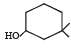Solution: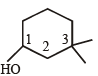IUPAC name –  3, 3-Dimethyl -1 cyclohexanol

QUESTION: 19

Which one of the following does not have sp2 hybridized carbon ?

Solution: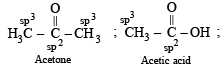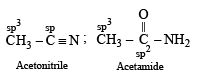QUESTION: 20

Which of the following will have a mesoisomer also?

Solution:

NOTE : The compounds containing two similar assymmetric C-atoms have plane of symmetry and exist in Meso form.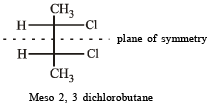QUESTION: 21

Rate of the reaction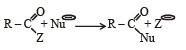is fastest when Z is

Solution:

Cl is the best leaving group among the given option.

QUESTION: 22

Amongst the following compounds, the optically active alkane having lowest molecular mass is

Solution:

Only 2- cylcopropyl butane has a chiral centre.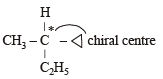QUESTION: 23

Consider the acidity of the carboxylic acids :

(a) PhCOOH

(b) o-NO2C6H4COOH

(c) p-NO2C6H4COOH

(d) m-NO2C6H4COOH

Which of the following order is correct ?

Solution:

In aroma tic acids presence of electron withdrawing substituent e.g. –NO2 disperses the negative charge of the anion and stablises it and hence increases the acidity of the parent benzoic acid.
Further o-isomer will have higher acidity than corresponding m and p isomers. Since nitro group at p-position have more pronounced electron withdrawing than –NO2 group at m-position hence the correct order is the one given above.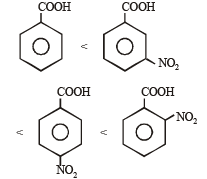QUESTION: 24

Which of the following is the strongest base ?

Solution:

Lone pair of electrons present on the  nitrogen of  benzyl amine is not involved  in resonance

QUESTION: 25

Which of the following compounds is not chiral?

Solution:

1-chloropentane is not chiral while others are chiral in nature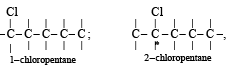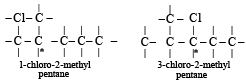QUESTION: 26

Due to the presence of an unpaired electron, free radicals are:

Solution:

Free radicals are electrically neutral, unstable and very reactive on account of the presence of odd electrons.

QUESTION: 27

The decreasing order of nucleophilicity among the nucleophiles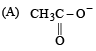(B) CH3O-

(C) CN-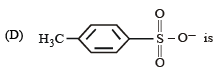Solution:

In moving down a group, the basicity and nucleophilicity are inversely related, i.e. nucleophilicity increases while basicity decreases. In going from left to right across a period, the basicity and nucleophilicity are directly related. Both of the characteristics decrease as the electronegativity of the atom bearing lone pair of electrons increases. If the nucleophilic centre of two or more species is same, nucleophilicity parallels basicity, i.e. more basic the species, stronger is its nucleophilicity.
Hence based on the above facts, the correct order of nucleophilicity will be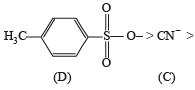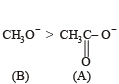QUESTION: 28

The reaction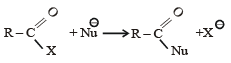is fastest when X is

Solution: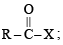when X is Cl the C–X bond is more polar and  ionic which leaves the compound more reactive for nucleophilic substitution reaction.

QUESTION: 29

The IUPAC name of the compound shown below is :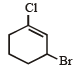Solution: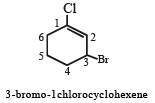QUESTION: 30

The increasing order of stability of the following free radicals is

Solution:

The order of stability of free radicals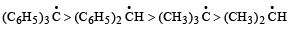The stabilisation of first two is due to resonance and last two is due to inductive effect.

QUESTION: 31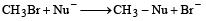The decreasing order of the rate of the above reaction with nucleophiles (Nu) A to D is

[Nu = (A) PhO, (B) AcO, (C) HO, (D) CH3O]

Solution:

TIPS/Formulae :
The stronger the acid, the weaker the conjugate base formed.
The acid character follows the order : CH3COOH > C6H5OH > H2O > CH3OH
The basic character will follow the order CH3COO < C6H5O < OH < CH3O

QUESTION: 32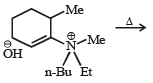The alkene formed as a major product in the above elimination reaction is

Solution:

Hofmann's rule : When theoretically more than one type of alkenes are possible in eliminations reaction, the alkene containing least alkylated double bond is formed as major product. Hence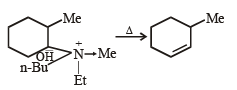NOTE : It is less stearically b-hydrogen is removed

QUESTION: 33

Incr easin g order of stabili ty among th e thr ee main conformations (i.e. Eclipse, Anti, Gauche) of 2-fluoroethanol is

Solution: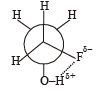Due to hydrogen bonding between H & F gauche conformation is most stable hence the correct order is

Eclipse, Anti, Gauche

QUESTION: 34

The IUPAC name of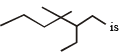Solution: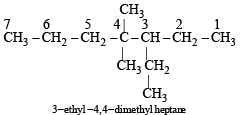QUESTION: 35

Which of the following molecules is expected to rotate the plane of plane-polarised light?

Solution:

NOTE : The organic compounds which have chiral carbon atom (a carbon atom attached to four different group or atoms and do not have plane of symmetry rotate plane polarised light.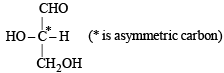QUESTION: 36

Presence of a nitro group in a benzene ring

Solution:

Nitro group is electron with drawing group, so it deactivates the ring towards electrophilic substitution.

QUESTION: 37

Which one of the following conformations of cyclohexane is chiral?

Solution:

Chiral conformation will not have plane of symmetry.
Since twist boat does not have plane of symmetry it is chiral.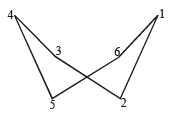QUESTION: 38

The absolute configuration of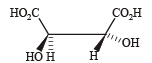Solution:

The absolute configuration is (R, R) (using priority rules to get the absolute configuration) So the correct answer is (b)

QUESTION: 39

The electrophile,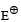attacks the benzene ring to generate the intermediate σ-complex. Of the following, which scomplex is lowest energy?

Solution:

In option (b) the complex formed is with benzene where as in other cases it is formed with nitrobenzene with – NO2 group in different position (o-, m-, p-).  The complex formed with nitrobenzene in any position of –NO2 group is less stable than the complex formed with benzene so the correct answer is (b) NOTE : The most stable complex has lowest energy.

QUESTION: 40

The correct decreasing order of priority for the functional groups of organic compounds in the IUPAC system of nomenclature is

Solution:

The correct order of priority for the given functional group is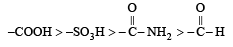QUESTION: 41

The IUPAC name of neopentane is

Solution: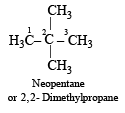QUESTION: 42

Arrange the carbanions,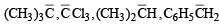in order of their decreasing stability :

Solution: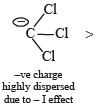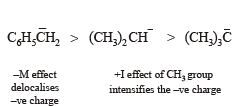QUESTION: 43

The alkene that exhibits geometrical isomerism is:

Solution: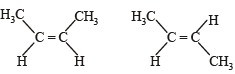QUESTION: 44

The number of stereoisomers possible for a compound of the molecular formula

CH3 – CH = CH – CH(OH) – Me is:

Solution: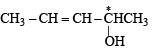exhibits both geometrical as well as optical isomerism.

cis - R     cis - S
trans - R  trans - S

QUESTION: 45

The correct order of in creasing basicity of the given conjugate bases (R = CH3) is

Solution:

The correct order of basicity is

RCOO- < CH ≡ C- < NH2 - < R-

QUESTION: 46

Out of the following, the alkene that exhibits optical isomerism is

Solution:

For a compound to show optical isomer ism, presence of chiral carbon atom is a necessary condition.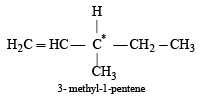QUESTION: 47

Identify the compound that exhibits tautomerism :

Solution: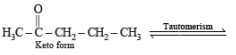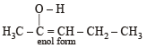QUESTION: 48

A solution of (–) – 1 – chloro –1– phenylethane in toluene racemises slowly in the presence of a small amount of SbCl5, due to the formation of :

Solution: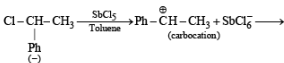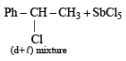QUESTION: 49

The order of stability of the following carbocations :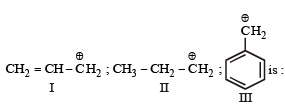Solution:

Higher stability of allyl and aryl substituted methyl carbocation is due to dispersal of positive charge due to resonance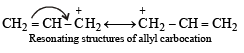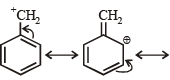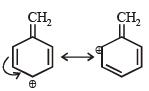Resonating structures of benzyl carbocation

whereas in alkyl carbocations dispersal of positive charge on different hydrogen atoms is due to hyper conjugation hence the correct order of stability will be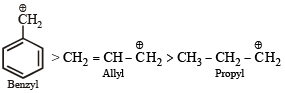QUESTION: 50

For the estimation of nitrogen, 1.4 g of an organic compound was digested by Kjeldahl method and the evolved ammonia was absorbed in 60 mL of M/10 sulphuric acid. The unreacted acid required 20 mL of M/10 sodium hydroxide for completeneutralization. The percentage of nitrogen in the compound is:

Solution: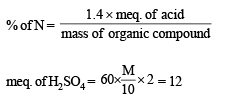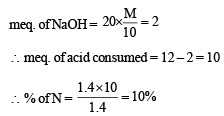QUESTION: 51

Which of the following compounds will exhibit geometrical isomerism ?

Solution: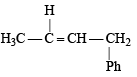1- Phenyl-2-butene the two groups around each of the doubly bonded carbon Because, all are different. This compound can show cis-and trans-isomerism.

QUESTION: 52

In Carius method of estimation of halogens, 250 mg of an organic compound gave 141 mg of AgBr. The percentage of bromine in the compound is :

(at. mass Ag =108; Br = 80)

Solution:

Mass of substance = 250 mg = 0.250 g
Mass of AgBr = 141 mg = 0.141 g
1 mole of AgBr = 1 g atom of Br
188 g of AgBr = 80 g of Br
∴ 188 g of AgBr contain bromine = 80 g

0.141 g of AgBr contain bromine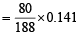This much amount of bromine present in 0.250 g of organic compound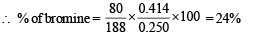QUESTION: 53

The absolute configuration of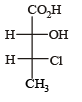is :

Solution: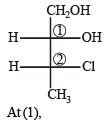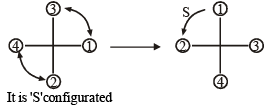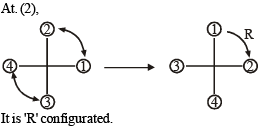QUESTION: 54

The distillation technique most suited for separating glycerol from spent-lye in the soap industry is :

Solution:

Spent-lye and glycerol are separated by distillation under reduced pressure.
Under the reduced pressure the liquid boil at low temperature and the temperature of decomposition will not reach. e.g. glycerol boils at 290°C with decomposition but at reduced pressure it boils at 180° C without decomposition.